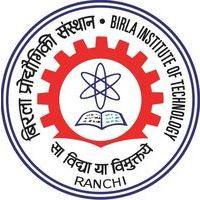GD Goenka, Admission Open for UG and PG Courses.

# BITS MCA Syllabus 2020, Section & Subject Wise Syllabus – Check Here

Latest Applications Open 2020:The Birla Institute of Technology, Mesra, Ranchi organizes the computer test online for getting admission into six semester MCA course provided by various campuses situated at Mesra, Jaipur, Lalpur, and Noida. The students will be selected by scores gained in the online test.

Candidates will be shortlisted for different programs on the bases of marks obtained in this online test.

The BITS MCA 2020 entrance exam will be held in June. The dates for is yet to be declared.

## Section A: Mathematics (60 Questions – Objective Type)

Algebra: Fundamental operations in Algebra, Expansion, factorization, quadratic equations, indices, logarithms, arithmetic, geometric and harmonic progressions, binomial theorem, permutations, and combinations, surds

• Set Theory: Sets and subsets, operations on sets, sequences, properties of integers, relations, and functions
• Matrix Algebra: Elementary transformations, the inverse of a matrix, rank, solution of simultaneous linear equations, eigenvalues and eigenvectors, quadratic forms
• Coordinate Geometry: Rectangular Cartesian coordinates, equations of a line, midpoint, intersections, etc., equations of a circle, distance formulae, the pair of straight lines, parabola, ellipse and hyperbola, simple geometric transformations such as translation, rotation, scaling.
• Calculus: Limit of functions, continuous functions, differentiation of function(s), tangents and normals, simple examples of maxima and minima, integration of function by parts, by substitution and by partial fraction, definite integral application to volumes and surfaces of frustums of a sphere, cone, cylinder, Taylor Series.
• Differential Equations: Differential equations of the first order and their solutions, linear differential equations with constant coefficients, homogeneous linear differential equations.
• Vectors: the Position vector, addition, and subtraction of vectors, scalar and vector products and their applications to simple geometrical problems and mechanics.
• Trigonometry: Simple identities, trigonometric equations, properties of triangles, the solution of triangles, height and distance, inverse function
• Probability and Statistics: Basic concepts of probability theory, averages, dependent and independent events, frequency distributions, and measures of dispersion, skewness, and kurtosis, random variable and distribution functions, mathematical expectations, binomial, Poisson, normal distributions, curve fitting, and the principle of least squares, correlation, and regression
• Linear Programming: Formulation of simple linear programming problems, basic concepts of graphical and simplex methods, revised simplex method, transportation and assignment problems, duality and integer programming

## Section B: Analytical Ability and Logical Reasoning: (20 questions – objective type)

Latest Applications For Various UG & PG Courses Open 2020:

1. Manav Rachna University, Haryana – 2020 UG & PG Admission Open. Apply Now
2. Bennett University (Times Group), Admission Open for 2020. Apply Now
4. Chandigarh University, Punjab 2020 Admission Open for all Courses. Apply Now
5. Sharda University, Admission Open 2020, Few Days left. Apply Now
6. MIT World Peace University, Admissions Open for All Courses 2019-20. Apply Now
7. St. Andrew’s Institute of Technology and Management, Admission open 2020. Apply Now
8. GD Goenka, Admission Open for UG & PG Courses. Apply Now

Questions in this section will test the logical reasoning, quantitative reasoning, and vision-spatial reasoning

## Section C: Computer Awareness: (20 questions – Objective Type)

• Computer Basics: Organization of a computer, Central Processing Unit (CPU), Structure of instructions in CPU, input/output devices, computer memory, memory organization, backup devices
• Data Representation: Representation of characters, integers, and fractions, binary and hexadecimal representations, Binary Arithmetic: Addition, subtraction, division, multiplication, 1’s and 2’s complement arithmetic, floating-point representation of numbers, normalized floating-point representation, Boolean algebra, truth tables, Venn diagrams
• Computer Architecture: Block structure of computers, communication between the processor and I / O devices, interrupts
• Computer Language: Assembly language and high-level language, Multiprogramming and time-sharing operating systems, Computer Programming in C.
• Flowchart and Algorithms
• Operating Systems: Evolution of operating systems, types of operating systems, functions of an operating system, modern operating systems

## Section D: English: (20 questions – Objective Type)

Latest Applications For Various UG & PG Courses Open 2020:

1. Manav Rachna University, Haryana – 2020 UG & PG Admission Open. Apply Now
2. Bennett University (Times Group), Admission Open for 2020. Apply Now
4. Chandigarh University, Punjab 2020 Admission Open for all Courses. Apply Now
5. Sharda University, Admission Open 2020, Few Days left. Apply Now
6. MIT World Peace University, Admissions Open for All Courses 2019-20. Apply Now
7. St. Andrew’s Institute of Technology and Management, Admission open 2020. Apply Now
8. GD Goenka, Admission Open for UG & PG Courses. Apply Now
• Use of articles and prepositions (fill in the blanks or correct use)
• Idioms and phrases
• Synonyms
• Expansion of an idea
• Sentence sequence (jumbled sentences)
• Completion of a sentence (with choices)
• Choice of the appropriate word to fill in the blanks (with options)

If you have any other Questions related to BITS MCA Syllabus 2020, you may ask your Queries by commenting below.

TagsManav Rachna UniversityMIT WPU